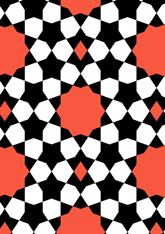# Ali Qoli Aqa mosque, Isfahandata16/F114

## Geometry

• The symmetry group of the tiling is 2*22 (cmm).
• All the internal angles of the constituent polygons are a multiple of 36°.
• Contains one regular two-pointed star polygon with vertex angle of 72°.
• Contains one regular five-pointed star polygon with vertex angle of 72°.
• Contains one regular 10-pointed star polygon with vertex angle of 108°.
• There are three non-regular reflective tiles (including one kite).
• The tiling satisfies the interlace condition and has no finite interlace and three infinite interlaces with straight cross-overs.
• The tiling is edge-to-edge.
• As drawn, contains about 225 polygons.

## References

Publications referenced:
1. Vol 3, Figure 114, page 175 (Ali Qoli Aqa mosque, Isfahan, Iran) from [iran] (1122AD, 515AH)